## Brocard AngleDefine the first Brocard Point as the interior point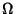of a Triangle for which the Angles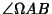,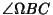, and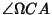are equal. Similarly, define the second Brocard Point as the interior pointfor which the Angles,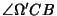, and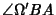are equal. Then the Angles in both cases are equal, and this angle is called the Brocard angle, denoted.

The Brocard angleof a Triangle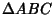is given by the formulas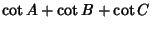(1)(2)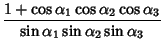(3)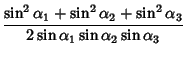(4)(5)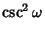(6)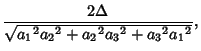(7)

whereis the Triangle Area,,, andare Angles, and,, andare side lengths.

If an Angle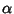of a Triangle is given, the maximum possible Brocard angle is given by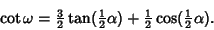(8)

Let a Triangle have Angles,, and. Then(9)

where(10)

(Le Lionnais 1983). This can be used to prove that(11)

(Abi-Khuzam 1974).

See also Brocard Circle, Brocard Line, Equi-Brocard Center, Fermat Point, Isogonic Centers

References

Abi-Khuzam, F. Proof of Yff's Conjecture on the Brocard Angle of a Triangle.'' Elem. Math. 29, 141-142, 1974.

Johnson, R. A. Modern Geometry: An Elementary Treatise on the Geometry of the Triangle and the Circle. Boston, MA: Houghton Mifflin, pp. 263-286 and 289-294, 1929.

Le Lionnais, F. Les nombres remarquables. Paris: Hermann, p. 28, 1983.